# Avoid Duplication in Serial Numbers in Excel

A friend called me and asked if there is a way to have serial numbers in such a way that they are no duplication in serial numbers in Excel.

Something as shown below: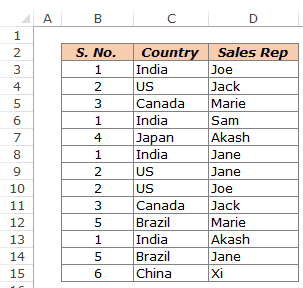He wanted that the serial number for India should be 1 wherever it occurs. Similarly, US is the 2nd country and should always have 2 as its serial number.

This got me thinking.

And here are the two ways that I could come up with to avoid duplication in serial numbers in Excel.

##### Method #1 – Using VLOOKUP Function

The first way is to use our beloved VLOOKUP function.

To do this, we first need to get a unique list of countries. Here are the steps to do that:

• Create a copy of the list of countries (copy paste it in the same worksheet or another worksheet).
• Select the copied data and go to Data –> Remove Duplicates. It will open the remove duplicate dialogue box.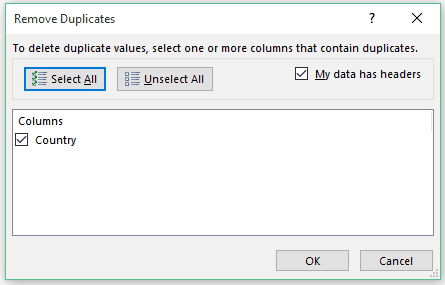• Make sure that the option – My data has headers is checked (in case your data has the header. Else uncheck it).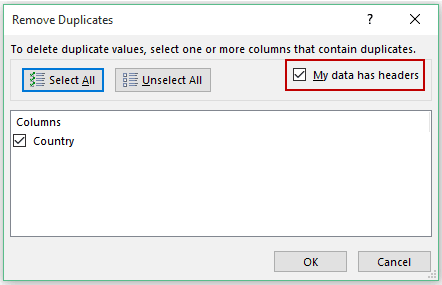• Select the column from which you want to remove ṭhe duplicates.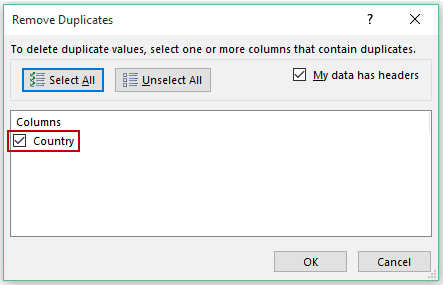• Click OK.
• That’s it. You will have a list of unique country names.
`See Also: The Ultimate Guide to Find and Remove Duplicates in Excel.`

Now assign the serial numbers to each country. Make sure these numbers entered to the right of the unique country list, as VLOOKUP can’t fetch data from the left of the lookup value.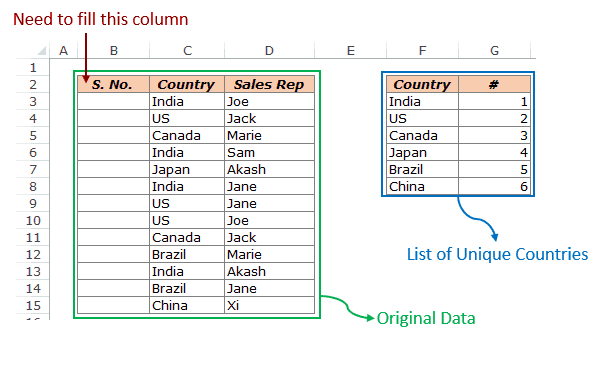In the cell, where you want the serial numbers (B3:B15), use the below VLOOKUP formula:

=VLOOKUP(C3,\$F\$3:\$G\$8,2,0)

This VLOOKUP formula takes the country name as the lookup value, checks for it in the data in F3:G8, and returns its serial number.

##### Method #2 – A Dynamic Formula

While the VLOOKUP method is a perfectly fine way of doing this, it is not dynamic.

So if I add a new country or change an existing country, this method would not work and you will have to repeat the entire process of method #1 again.

Here is a formula that makes it dynamic:

=IF(COUNTIF(\$C\$3:\$C4,\$C4)=1,MAX(\$B\$3:\$B3)+1,INDEX(\$B\$3:\$C\$18,MATCH(\$C4,\$C\$3:\$C4,0),1))

To use this formula, you need to manually enter 1 in the first cell, and the above formula in all the other remaining cells.

How it works:

It uses an IF function that checks the number of times a country has occurred prior to that row. If the country name occurs for the first time, the count is 1 and the condition is TRUE, and if the country name has occurred earlier as well, the count is more than 1 and the condition is FALSE.

• When the condition is TRUE:

=MAX(\$B\$3:\$B3)+1

If the value is TRUE, which means that the country name is appearing for the first time, it identifies the maximum value of serial number till then and adds 1 to it to give the next serial number value.

• When Value if FALSE:

=INDEX(\$B\$3:\$C\$18,MATCH(\$C4,\$C\$3:\$C4,0),1)

If the country has already occurred earlier, this formula goes to the cell where it appears first and returns the serial number of the first occurrence of that country.

Download the Example File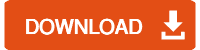You May Also Like the Following Excel Tutorials:FREE EXCEL BOOK

## Get 51 Excel Tips Ebook to skyrocket your productivity and get work done faster

### 2 thoughts on “Avoid Duplication in Serial Numbers in Excel”

1. Need a serial number

2. I need a serial number